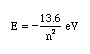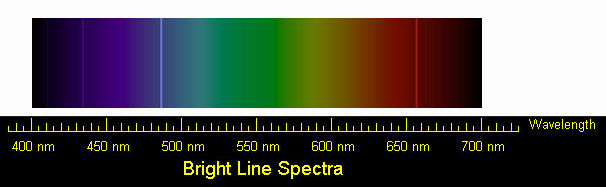# National Aeronautics and Space Administration

## Goddard Space Flight Center## Solution for Student Worksheet: Energy Levels in the Atom

### Solution for Calculate the Energy! Student Worksheet

Neils Bohr numbered the energy levels (n) of hydrogen, with level 1 (n=1) being the ground state, level 2 being the first excited state, and so on. Remember that there is a maximum energy that each electron can have and still be part of its atom. Beyond that energy, the electron is no longer bound to the nucleus of the atom and it is considered to be ionized. In that case n approaches infinity.

The equation for determining the energy of any state (the nth) is as follows:Because the energy is so small, the energy is measured in electron-volts, designated by "eV". 1 eV = 1.6 x 10-19 J.

1. Using the above expression, calculate the energy of the first excited state. Your answer will be negative. This signifies that the electron is bound to the atom (as opposed to being a free electron).

For the first excited state, n=2. Using this in the above equation gives E = -3.40 eV

2 . Use the above expression to find the energy of the photon released when an electron around a hydrogen atom moves from the 4th to the 2nd level.

The energy of the photon is found by computing the difference
in the energies of the fourth (n=4) and second (n=2) levels

E = -13.6/42 - (-13.6/22)
E = -0.85 + 3.40
E = 2.55 eV

3. Now use the above expression to find the energy of the photon released when a free electron is captured to the 2nd level.

We represent a free electron by assigning it an infinite n. Hence, its energy is zero.
The energy of the photon emitted by a free electron captured to the n=2 level is thus
E = 0 - (-13.6/22) = 3.4 eV

4. Use the relationship between a photon's energy and its wavelength to calculate the wavelength of the photon emitted in question 2.

From the Calculation Investigation, we learned that energy and wavelength are related through E = h c / l.
We can solve this for the wavelength, l = h c / E. where h = 6.626 x 10-34J-s, and c = 3 x 108 m/s.
We convert our energy E= 2.55 ev into Joules using 1 eV = 1.6x10-19 J. This gives an energy of E = 4.08 x 10-19 J.

We then find a wavelength of
l = ((6.626 x 10-34) x (3 x 108)) / (4.08 x 10-19)
l = 4.87 x 10-7 m.
OR, using 1 nm = 1 x 10-9 m,

l = 487 nm.

5. Compare the wavelength for this transition with the lab spectrum of hydrogen below.

The transition is the bright blue line, just to the left of the center.A service of the High Energy Astrophysics Science Archive Research Center (HEASARC), Dr. Alan Smale (Director), within the Astrophysics Science Division (ASD) at NASA/GSFC